# Force

Newton's Laws of Motion

First Law of Motion

An object continues to be in its state of rest or uniform motion, until and unless an external unbalanced force acts on it.

Second Law of Motion

Force acting on an object is directly proportional to the rate of change of Momentum with respect to time.

Third Law of Motion

For every action, there is an equal and opposite reaction. This reaction is limiting and acts in the opposite direction as long as it is capable of withstanding the force.

Force

Force = Mass x Acceleration

Forces in Nature

Gravitational Force

Force of attraction between two bodies having mass.

Electromagnetic Force

Forces involved in electric and magnetic fields.

Strong Nuclear Force:

A nucleus contains protons and neutrons. Protons being positively charged exert repulsive force on each other. But, this force is not enough to break the nucleus. This is because there exists a strong interaction inside the nucleus. This is called the strong nuclear force.

Weak Nuclear Force:

Forces associated with radioactive reactions are called Weak Nuclear Forces.

Law of Conservation of Momentum

The law of conservation of momentum states that the total momentum of a system of objects is always conserved.

Impulse is the amount of force acting on a body in given time. Impulse and Momentum have the same dimensions.

𝐼𝑚𝑝𝑢𝑙𝑠𝑒=𝐽=𝐹.𝑡

Frame of Reference

A Frame of reference is a fixed co-ordinate system, with respect to which the position and motion of an object is determined.

Inertial

Inertial frame of reference is a frame of reference in which Newton's First Law of Motion holds good. i.e. it is an unaccelerated frame of reference.

Non-Inertial

Non-Inertial Frame of reference is a frame of reference in which Newton's First Law of Motion does not hold good. i.e. It is an accelerated frame of reference. Since it is an accelerated frame of reference, even if no force is acting on the object, the object will be set in motion . e.g. A person sitting in train.

Conservative and Non Conservative Force

The Forces that conserve Total Energy, Kinetic Energy and Momentum are called as Conservative Forces.

The Forces that conserve Total Energy and Momentum but not Kinetic Energy are called Non Conservative Forces.

Moment of Force

Moment of Force = Force x Moment arm

Moment Arm is the distance through which the Force is acting.

Couple & Torque

Torque = Force x Distance between the two forces.

Centre of Mass

The fixed point of an object at which the entire mass of the object is supposed to be concentrated is called Centre of Mass.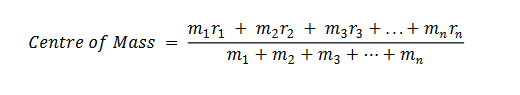Centre of Gravity

The fixed point through which the entire weight of the object is supposed to act in the downward direction is called as Centre of Gravity.

Conditions of Equilibrium of Forces

The resultant force should be 0; i.e. it should not produce acceleration. Thus, the vector sum of all forces acting on the body should be 0.

If the Forces in Equilibrium prevent rectilinear motion, it is called as translational equilibrium. If the forces in equilibrium prevent rotational motion, it is called rotational equilibrium.

Important for solving Problems:

Apparent weight of a man inside a lift:

(a) The lift possesses zero acceleration: W = mg

(b) The lift moving upward with an acceleration a: W = mg + ma

(c) The lift moving downward with an acceleration a: W = mg –ma

Connected motion (Pulley problem):

Driven body moving vertically: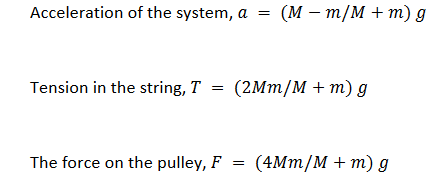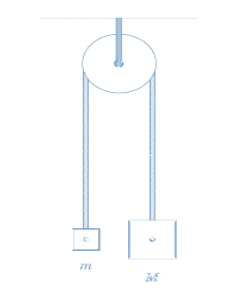Driven body moving horizontally: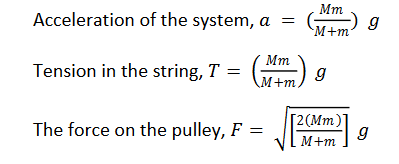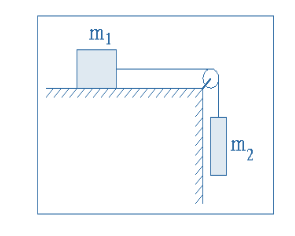Post By : Ravindra Yadav 03 Nov, 2019 1684 views Physics